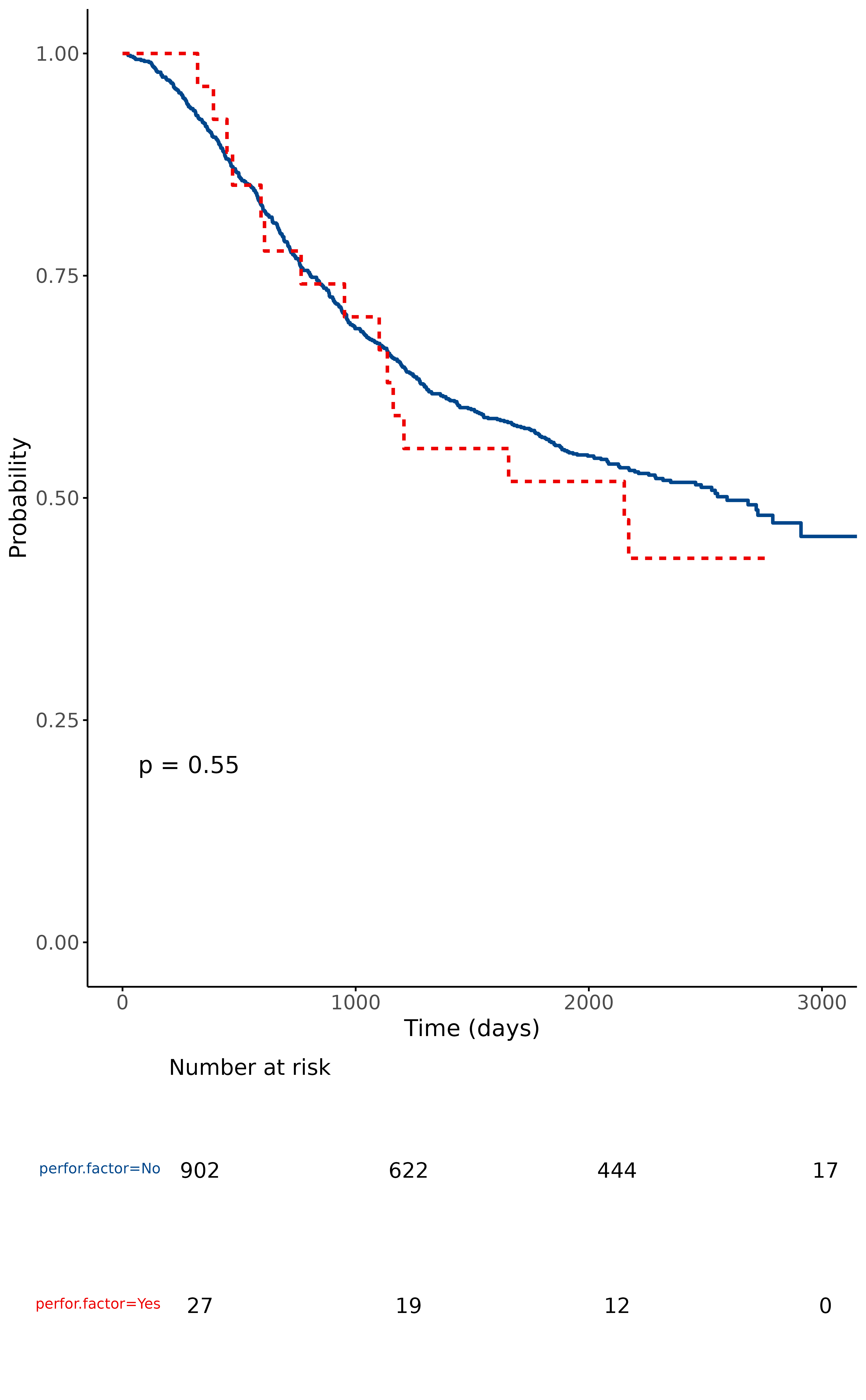Produce a survival curve plot and number-at-risk table using survminer::ggsurvplot and finalfit conventions.

surv_plot(.data, dependent, explanatory, ...)

## Arguments

.data

Dataframe.

dependent

Character vector of length 1: Survival object of the form Surv(time, status).

explanatory

Character vector of max length 2: quoted name(s) of explanatory variables.

...

Arguments passed to ggsurvplot.

## Value

Returns a table and plot produced in ggplot2.

Other finalfit plot functions: coefficient_plot(), ff_plot(), hr_plot(), or_plot()

## Examples

library(finalfit)
library(dplyr)

# Survival plot
data(colon_s)
explanatory = c("perfor.factor")
dependent = "Surv(time, status)"
colon_s %>%
surv_plot(dependent, explanatory, xlab="Time (days)", pval=TRUE, legend="none")